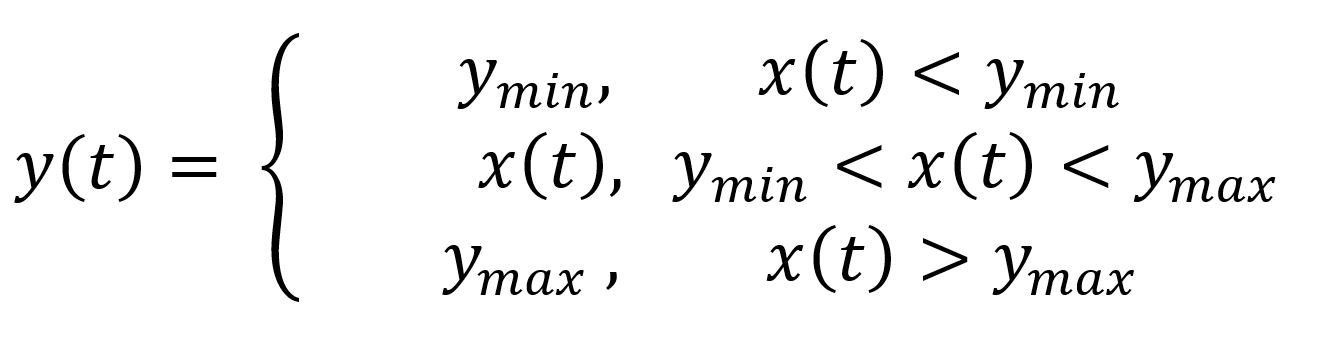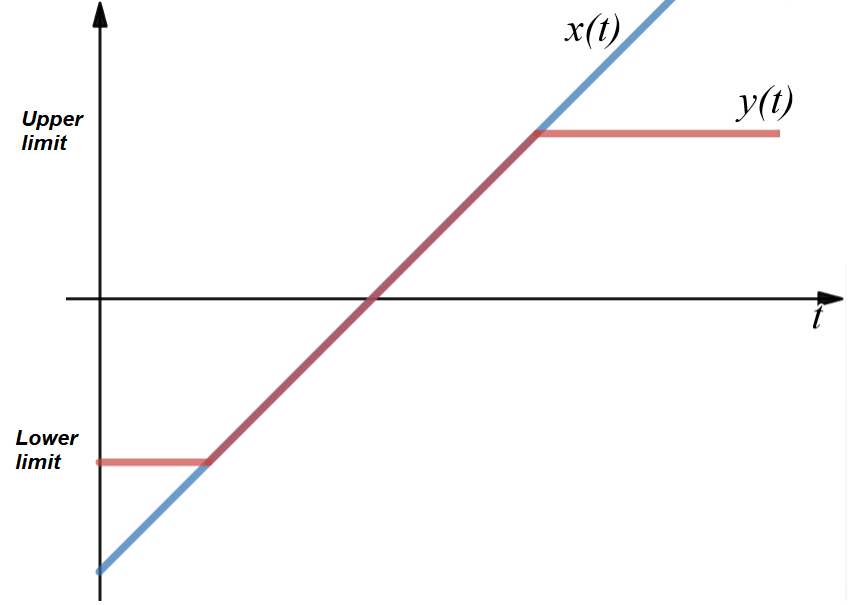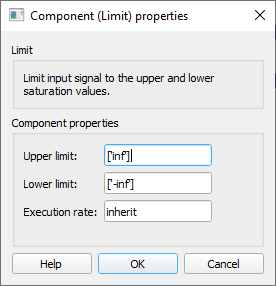# Limit

Description of the Limit component in Schematic Editor, which limits the input signal to upper and lower limits.

## Description

The Limit component limits the input signal to the upper and lower limits defined in the component properties.

The function of the Limit component can be represented with the equation and the figure presented below.Where ymin and ymax are the upper and the lower threshold values.

Figure 2 shows the output signal y(t) of the limit component for a given input signal x(t).Figure 2. Output of the Limit component for a given input signal

The values ‘inf’ and ‘-inf’ indicate that there are no limitations.

## Ports

• Input (in)
• Original input signal.
• Supported types: uint, int and real.
• Vector support: yes.
• Output (out)
• Limited input signal.
• Supported types: uint, int and real.
• The output type is inherited from the input signal.
• Vector support: yes.
• The vector length is inherited from the input signal or calculated using property value lengths. If the property is defined as a vector, the output will be a vector of the same length.

## Properties• Upper limit
• Type in the input upper limits. The value can be a scalar or a vector value. If the value is scalar, the operation is performed element-wise on the input vector.
• Lower limit
• Type in the input lower limits. The value can be a scalar or a vector value. If the value is scalar, the operation is performed element-wise on the input vector.
• Execution rate
• Type in the desired signal processing execution rate. This value must be compatible with other signal processing components of the same circuit: the value must be a multiple of the fastest execution rate in the circuit. There can be up to four different execution rates, but they must all be multiple of the basic simulation timestep. To specify the execution rate, you can use either decimal (e.g. 0.001) or exponential values (e.g. 1e-3) in seconds. Alternatively, you can type in ‘inherit’ in which case the component will be assigned execution rate based on the execution rate of the components it is receiving input from.